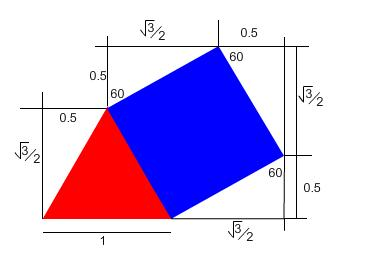#### You may also like### Be Reasonable

Prove that sqrt2, sqrt3 and sqrt5 cannot be terms of ANY arithmetic progression.### Good Approximations

Solve quadratic equations and use continued fractions to find rational approximations to irrational numbers.### Rational Roots

Given that a, b and c are natural numbers show that if sqrt a+sqrt b is rational then it is a natural number. Extend this to 3 variables.

##### Age 16 to 18 ShortChallenge Level

This is quite interesting. The key idea is based on this picture:Once you get the hang of it you can easily count your way around any path. Paths can be arranged so that the irrational part cancels out by having equal numbers going up/down or equal numbers going left/right.

Dapeng Wang from Claremont School sent in a good solution to this problem. His idea was to analyse different possible combinations of two shapes. We present his basic idea but formulate it slightly differently below.

Suppose we have constructed a Munchkin road. The first tile is required to have a corner at the point $(0,0)$. It is clear when we think about it that the destination of the road (i.e. the top/bottom vertex of the last triangle) can be reached by tracing a path from $(0,0)$ along edges of the shapes that make up the road. We set up an induction argument. $(0,0)$ is obviously of the form $(\frac{a+b\sqrt{3}}{2},\frac{c+d\sqrt{3}}{2})$. Now suppose we choose a path from $(0,0)$ to the destination of the road. Pick a vertex on this path, which has coordinates $(x,y)$ say. All edges in the road have length $1$.Bearing in mind that the triangles are equilateral, it is clear that the angle to the horizontal of any edge must be a multiple of $30^{\circ}$. Therefore the next vertex in the path must be one of $(x\pm 1,y)$, $(x,y\pm 1)$, $(x\pm\frac{1}{2},y\pm\frac{\sqrt{3}}{2})$ or $(x\pm\frac{\sqrt{3}}{2},y\pm\frac{1}{2})$. By induction, then, any vertex on the path, and in particular the destination of the path, is of the form$(\frac{a+b\sqrt{3}}{2},\frac{c+d\sqrt{3}}{2})$.